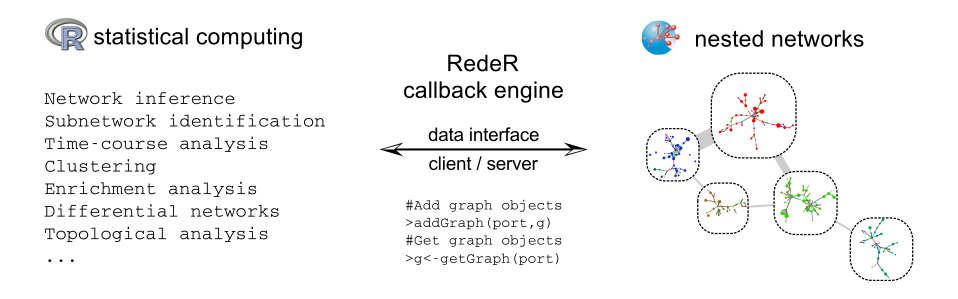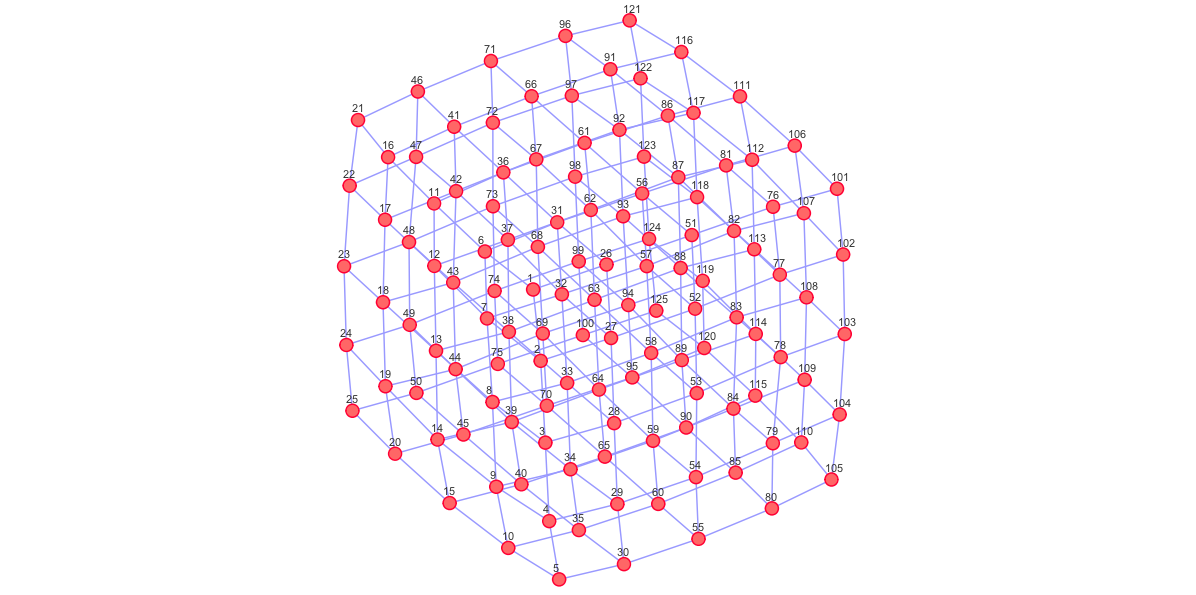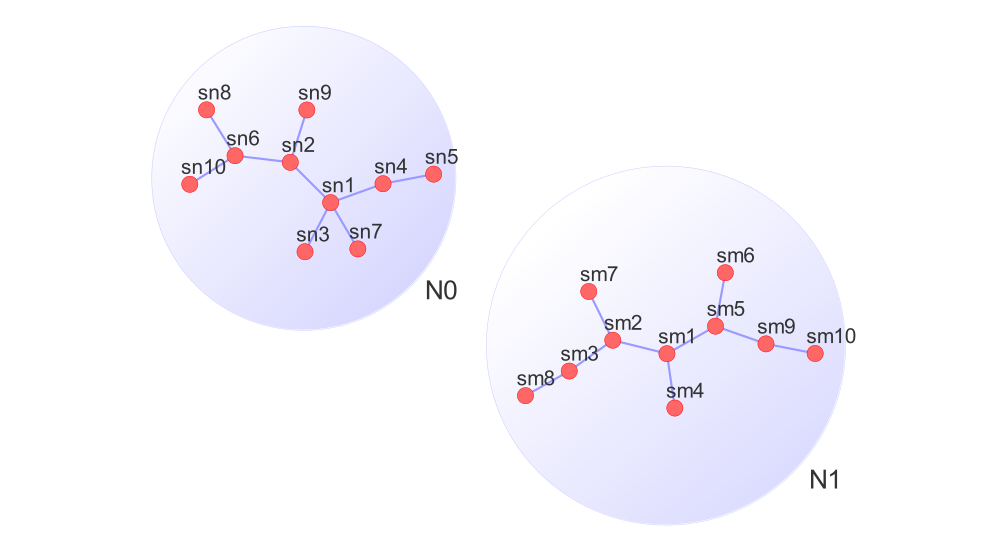# 1 Overview

RedeR is an R-based package combined with a Java application for interactive network visualization and manipulation. It implements a callback engine by using a low-level R-to-Java interface to run complementary methods. In this sense, RedeR takes advantage of R to run robust statistics, while the R-to-Java interface bridge the gap between network analysis and visualization.

The package is designed to deal with three key challenges in network analysis. Firstly, biological networks are modular and hierarchical, so network visualization needs to take advantage of such structural features. Secondly, network analysis relies on statistical methods, many of which are already available in resources like CRAN or Bioconductor. However, the missing link between advanced visualization and statistical computing makes it hard to take full advantage of R packages for network analysis. Thirdly, in larger networks user input is needed to focus the view of the network on the biologically relevant parts, rather than relying on an automatic layout function (additional information is available at Castro et al. (2012)). The design of the software is depicted from Figure 1. Complex graphs with many attributes can be transferred from-and-to R using `addGraph` and `getGraph` functions.Figure 1. Schematic representation of RedeR calls. In the low-level interface, the Apache server (Apache Software Foundation" 2010) is used to link R to Java.

# 2 Quick Start

## 2.1 Main callback methods

The first step is to build the server port, which will be required for all graph calls. By default the constructor `RedPort` should set all details:

``library(RedeR)``
``````## ***This is RedeR 1.42.0! For a quick start, please type 'vignette('RedeR')'.
##    Supporting information is available at Genome Biology 13:R29, 2012,
##    (doi:10.1186/gb-2012-13-4-r29).``````
``rdp <- RedPort() ``

Next, launch the RedeR interface using the `calld` function:

``calld(rdp)``

Then the method `addGraph` can be used to send R graphs to the application. For example, the following chunk sends an igraph object to RedeR (Fig. 2):

``````library (igraph)
g1 <- graph.lattice(c(5,5,5))Figure 2. A toy example added to RedeR by the `addGraph` function.

Conversely, RedeR graphs can be transferred to R and wrapped into igraph objects:

``````g2 <- getGraph(rdp)
resetd(rdp)``````

The interface accepts additional graph attributes, as for example edge direction, edge width, edge weight, node shape, node size, node color, etc. In order to pass these extensible features the attributes must be provided in a valid syntax (see `getGraph` and `addGraph` specification for additional details).

Another strategy is to wrap graphs into containers and then send it to the Java application. Next, the subgraphs `g3`and `g4` are assigned to different nested structures (Fig. 3).

``````g3 <- barabasi.game(10)
g4 <- barabasi.game(10)
V(g3)\$name<-paste("sn",1:10,sep="")
V(g4)\$name<-paste("sm",1:10,sep="")
addGraph(rdp, g3, isNest =TRUE, gcoord=c(25,25), gscale=50)
addGraph(rdp, g4, isNest =TRUE, gcoord=c(75,75), gscale=50)``````Figure 3. Nested graphs in RedeR using the command `addGraph`.

In this case, the subgraphs can be handled apart from each other. For example, the following chunk selects all nodes assigned to the container “N0” and then gets back the subgraph (the selection step can also be done interactively).

``````selectNodes(rdp,"N0")
g5 <- getGraph(rdp, status= "selected")
resetd(rdp)``````

As a suggestion, try some RedeR features in the Java side (e.g. open samples s2 or s3 in the main panel and enjoy the dynamic layout options!).

## 2.2 Interactive work

The next chunk generates a scale-free graph according to the Barabasi-Albert model (Csardi and Nepusz 2006) and sends the graph to RedeR without any layout information.

``````g6 <- barabasi.game(500)
``relax(rdp,p2=400,p5=30,ps=T)``